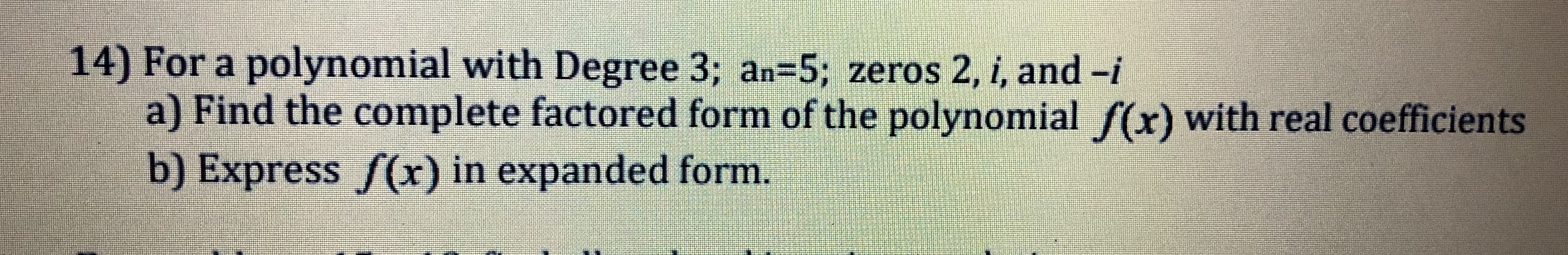# 14) For a polynomial with Degree 3; an-5; zeros 2, i, and -ia) Find the complete factored form of the polynomial f(x) with real coefficientsb) Express f(x) in expanded form

Question
28 viewshelp_outlineImage Transcriptionclose14) For a polynomial with Degree 3; an-5; zeros 2, i, and -i a) Find the complete factored form of the polynomial f(x) with real coefficients b) Express f(x) in expanded form fullscreen
check_circle

Step 1

Given:

Degree of the polynomial = 3

Real coefficient an = 5

Zeros of the polynomial are 2, i and -i.

Step 2

(a)

Given that the degree is 3 and the real coefficient is 5. The zeros are 2, i and -i.

Step 3

(b)

The expanded form i...

### Want to see the full answer?

See Solution

#### Want to see this answer and more?

Solutions are written by subject experts who are available 24/7. Questions are typically answered within 1 hour.*

See Solution
*Response times may vary by subject and question.
Tagged in

### Polynomials